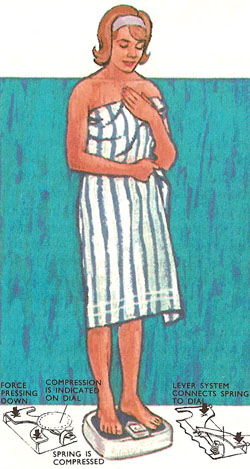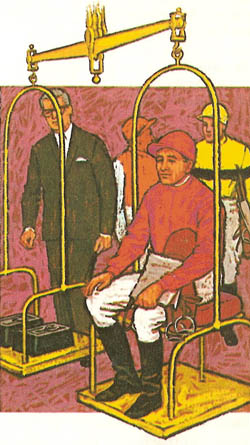A# massThe measurement of weight. This is the force with which a body pushes down on, for example, a spring. The amount the spring is compressed is measured by a system of levers.The measurement of mass. The mass of a jockey is exactly balanced by the masses placed on the other pan. Equal pulls are exerted on both pans irrespective of the size of the gravitational field.

In general, mass is the amount of matter in a body. Mass can be defined more precisely in terms of how difficult it is to change a body's state of motion or how great is the body's gravitational effect on other objects. The first of these is called inertial mass (see inertia) and is given by the factor m in Newton's second law F = ma (see Newton's laws of motion). The second is called gravitational mass and is the mass corresponding to an object's weight in a local gravitational field – the m in F = mg for an object on or near the Earth. According to all experiments, the values for m arising from these two definitions are identical.

Einstein's mass-energy relationship shows that mass and energy are interchangeable. For this reason, the law of conservation of mass in classical physics has been extended to become the law of conservation of mass and energy.

The SI unit of mass is the kilogram.

## The difference between mass and weight

Mass and weight are two of the most confused quantities in science. For example, we might say that if a bag contains 10 kilograms of potatoes then it "weighs" 10 kilograms, when, in fact, it's really the mass that we're talking about. The confusion is made worse by the fact that the scales (such as bathroom scales) on which we usually weigh things, including ourselves, are calibrated in kilograms or pounds – both units of mass. Take our bag of potatoes, for example. When placed on a scale, the needle moves around to 10 kilograms. The spring inside the scale offers an upward pull to the scale pan and its contents in an effort to stop itself from stretching. Gravitational forces acting on the potatoes try to pull them "down" (i.e., toward the center of the Earth). The spring extends until these two opposing forces exactly balance each other and then the weight can be read off a measure of the extension. The spring is measuring the size of the force trying to extend it, reflecting the fact that weight is a kind of force, so that (in SI units) the reading should really be in newtons.

The downward pull of the potatoes depends on two things: the amount of matter contained in the potatoes (their mass) and the gravitational pull of the Earth. Unless bits are chopped of the potatoes their mass stays the same, but the Earth's gravitational pull varies depending on location. For example, a stone dropped down a well will have an increased velocity of about 9.8 meters per second for every second it falls. But if the same stone were to fall from the same height as a satellite in orbit it's increase in velocity per second would be much less. This is because the stone would be further away from the Earth and thus less strongly affected by its gravitational field. Using a spring instrument, our bag of potatoes would weigh slightly less on a mountain top than at sea level.

But the mass of an object doesn't depend on location. A block of lead, for instance, is composed of a particular number of atoms. Each lead atom (of the commonest isotope) has 82 protons, 126 neutrons, and 82 electrons, and has a constant mass. It doesn't matter if the block of lead is down a well, up a mountain, or on the surface of the Moon, provided nothing has been chopped off it or stuck on it, it has the same mass.

Because of this, mass has to be measured using an instrument that will give the same answer wherever it is used. The beam balance is an example of such an instrument. The object of unknown mass is placed on the left hand balance pan. The pan drops and the beam tilts because their is nothing on the other pan to balance it. Metal "weights" (badly named – they should be called masses!) are placed on the other pan until a balance is achieved – mass is balanced against mass. When the balance is taken to a place where the gravitational field is less intense, there is less pull on the object, but also equally less pull on the 'weights in the other pan, and the same result is obtained.

The chemical balance is used for measuring masses to four decimal places. The weights must be treated with great care. If they become chipped, they lose some mass. If they are picked up with the fingers, grease from the hands attracts dirt and there is an increase in mass. They should always be handled with tweezers. This is one of the reasons why some of the more accurate balances are handled by remote control.